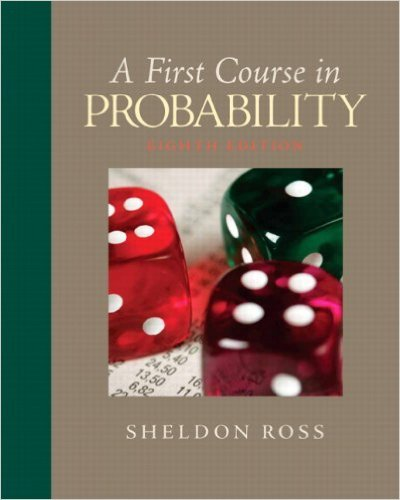×
×

# Seven balls are randomly withdrawn from an urnthatISBN: 9780136033134 163

## Solution for problem 35 Chapter 2

First Course in Probability | 8th Edition

• Textbook Solutions
• 2901 Step-by-step solutions solved by professors and subject experts
• Get 24/7 help from StudySoup virtual teaching assistantsFirst Course in Probability | 8th Edition

4 5 1 322 Reviews
13
5
Problem 35

Seven balls are randomly withdrawn from an urnthat contains 12 red, 16 blue, and 18 green balls.Find the probability that(a) 3 red, 2 blue, and 2 green balls are withdrawn;(b) at least 2 red balls are withdrawn;(c) all withdrawn balls are the same color;(d) either exactly 3 red balls or exactly 3 blue ballsare withdrawn.

Step-by-Step Solution:
Step 1 of 3

Color Coding: Topics MINITAB Examples STT 351 Notes Normal −1 2 f x = 1 e 2 x ( ) 2Π ­inf < x < inf √ To find probabilities between two points (a,b] we have to integrate this function between a,b. Using MINITAB: 1) Calc 2) Probability distribution 3) Normal (mean = 0, Standard deviation = 1) 4) Select cumulative...

Step 2 of 3

Step 3 of 3

##### ISBN: 9780136033134

Unlock Textbook Solution